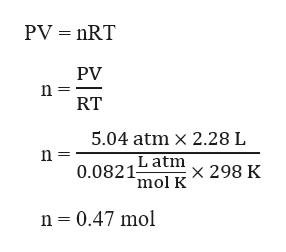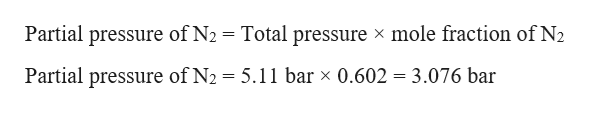# A student has a 2.28 L bottle that contains a mixture of O2 , N2 , and CO2 with a total pressure of 5.11 bar at 298 K . She knows that the mixture contains 0.283 mol N2 and that the partial pressure of CO2 is 0.289 bar. Calculate the partial pressure of O2.?O2=__________________________ bar

Question
669 views

A student has a 2.28 L bottle that contains a mixture of O2 , N2 , and CO2 with a total pressure of 5.11 bar at 298 K . She knows that the mixture contains 0.283 mol N2 and that the partial pressure of CO2 is 0.289 bar. Calculate the partial pressure of O2.

?O2=__________________________ bar

check_circle

Step 1

Given,

Volume, V = 2.28 L

Temperature, T = 298 K

Total pressure, P = 5.11 bar = 5.04 atm   (1 bar = 1/1.013 atm)

Moles of N2 = 0.283 mol

Partial pressure of CO2 = 0.289 bar

The total no. of moles of the mixture can be calculated using the ideal gas equation as:help_outlineImage TranscriptionclosePV nRT PV RT 5.04 atm x 2.28 L n L atm mol K 0.0821 x 298 K n 0.47 mol fullscreen
Step 2

The mole fraction of N2 can be calculated as:

Step 3

Partial pressure of N2 can be...help_outlineImage TranscriptionclosePartial pressure of N2 = Total pressure x mole fraction of N2 Partial pressure of N2 = 5.11 bar x 0.602 = 3.076 bar fullscreen

### Want to see the full answer?

See Solution

#### Want to see this answer and more?

Solutions are written by subject experts who are available 24/7. Questions are typically answered within 1 hour.*

See Solution
*Response times may vary by subject and question.
Tagged in

### Chemistry